Author: Oscar Cronquist Article last updated on August 26, 2021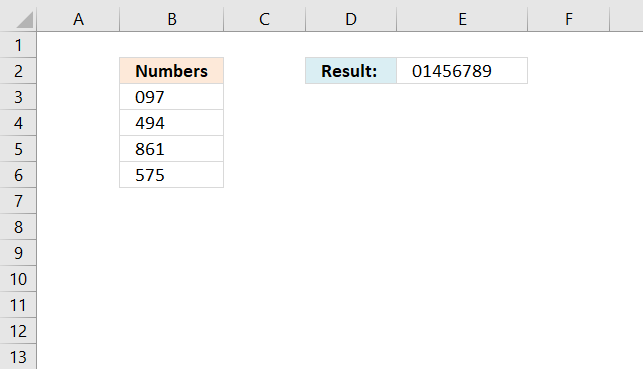This article demonstrates a formula that filters unique distinct single digits from a cell range containing numbers. Cell range B3:B6 contains three numbers in each cell.

The formula in cell E2 extracts all single digits from cell range B3:B6, however, sorted from small to large and only one instance of each digit.

Numbers 2 and 3 are not shown in cell E2 because they don't exist in cell range B3:B6. Note, it is not required to have three digits in each cell in cell range B3:B6. You can have as many as you want.

Find and Sort
I have question, in range A1,A2,A3,A4 contain 097 494 861 575.
What is the formula in excel if the result that i want is 01456789

Formula in cell E2:

=LET(x, {0,1,2,3,4,5,6,7,8,9}, TEXTJOIN("", TRUE, IF(MMULT(TRANSPOSE(ROW(B3:B6)^0), ISNUMBER(SEARCH(x, B3:B6))*1), x, "")))

This formula contains two functions that are only available for Excel subscribers. This formula will not work in earlier Excel versions.

Update 8/26/2021 - Smaller formula:

=LET(x, CONCAT(B3:B6), TEXTJOIN(, TRUE, SORT(UNIQUE(MID(x, SEQUENCE(LEN(x)), 1)))))

### Explaining formula in cell E2

#### Step 1 - Find search values in cell range

The SEARCH function allows you to search cell values and if a given text string is found a number is returned which represents the character position.

SEARCH(find_text, within_text, [start_num])

For example if 1 is returned the cell value begins with the text string.

The great thing about the SEARCH function is that it lets you search for multiple text strings, this makes the function return an array of numbers instead of a single number.

SEARCH({0, 1, 2, 3, 4, 5, 6, 7, 8, 9}, B3:B6)

becomes

SEARCH({0, 1, 2, 3, 4, 5, 6, 7, 8, 9}, {"097";494;861;575})

Note that the search strings are using the comma as a delimiting character and the the within_text argument uses semicolons as a delimiting character.

and returns

{1, #VALUE!, #VALUE!, #VALUE!, #VALUE!, #VALUE!, #VALUE!, 3, #VALUE!, 2;#VALUE!, #VALUE!, #VALUE!, #VALUE!, 1, #VALUE!, #VALUE!, #VALUE!, #VALUE!, 2;#VALUE!, 3, #VALUE!, #VALUE!, #VALUE!, #VALUE!, 2, #VALUE!, 1, #VALUE!;#VALUE!, #VALUE!, #VALUE!, #VALUE!, #VALUE!, 1, #VALUE!, 2, #VALUE!, #VALUE!}

The comma is a column delimiting character and the semicolon is a row delimiting character, if I enter the above array in cell C3 the formula expands automatically to cell range C3:L6. This is called spilling and is a new Excel 365 feature.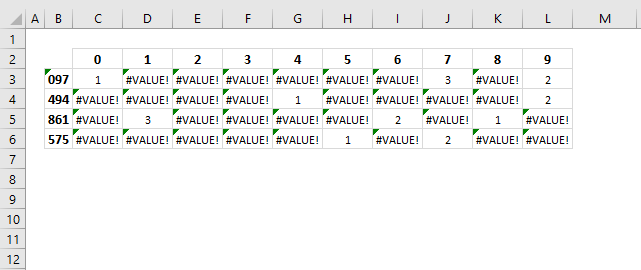The image above shows the find_text argument in row 2, the within_text in column B and the array in cell range C3:L6. Number 0 is found in the first value 097 in character position 1.

#### Step 2 - Identify non-error values

The ISNUMBER function lets you check if a cell value is a number, it returns the boolean value TRUE if a number and FALSE for everything else even error values.

ISNUMBER(SEARCH({0, 1, 2, 3, 4, 5, 6, 7, 8, 9}, B3:B6))

becomes

ISNUMBER({1, #VALUE!, #VALUE!, #VALUE!, #VALUE!, #VALUE!, #VALUE!, 3, #VALUE!, 2;#VALUE!, #VALUE!, #VALUE!, #VALUE!, 1, #VALUE!, #VALUE!, #VALUE!, #VALUE!, 2;#VALUE!, 3, #VALUE!, #VALUE!, #VALUE!, #VALUE!, 2, #VALUE!, 1, #VALUE!;#VALUE!, #VALUE!, #VALUE!, #VALUE!, #VALUE!, 1, #VALUE!, 2, #VALUE!, #VALUE!})

and returns

{TRUE, FALSE, FALSE, FALSE, FALSE, FALSE, FALSE, TRUE, FALSE, TRUE;FALSE, FALSE, FALSE, FALSE, TRUE, FALSE, FALSE, FALSE, FALSE, TRUE;FALSE, TRUE, FALSE, FALSE, FALSE, FALSE, TRUE, FALSE, TRUE, FALSE;FALSE, FALSE, FALSE, FALSE, FALSE, TRUE, FALSE, TRUE, FALSE, FALSE}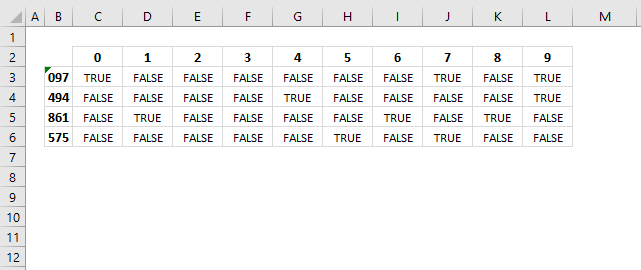This step is required, the MMULT function, which we will use in step 4, can't process error values.

#### Step 3 - Convert boolean values to their numerical equivalents

Boolean value TRUE is the same thing as 1 and FALSE is 0 (zero). It is easy to convert boolean values simply multiply with 1.

ISNUMBER(SEARCH({0, 1, 2, 3, 4, 5, 6, 7, 8, 9}, B3:B6))*1

becomes

{TRUE, FALSE, FALSE, FALSE, FALSE, FALSE, FALSE, TRUE, FALSE, TRUE;FALSE, FALSE, FALSE, FALSE, TRUE, FALSE, FALSE, FALSE, FALSE, TRUE;FALSE, TRUE, FALSE, FALSE, FALSE, FALSE, TRUE, FALSE, TRUE, FALSE;FALSE, FALSE, FALSE, FALSE, FALSE, TRUE, FALSE, TRUE, FALSE, FALSE}*1

and returns

{1, 0, 0, 0, 0, 0, 0, 1, 0, 1;0, 0, 0, 0, 1, 0, 0, 0, 0, 1;0, 1, 0, 0, 0, 0, 1, 0, 1, 0;0, 0, 0, 0, 0, 1, 0, 1, 0, 0}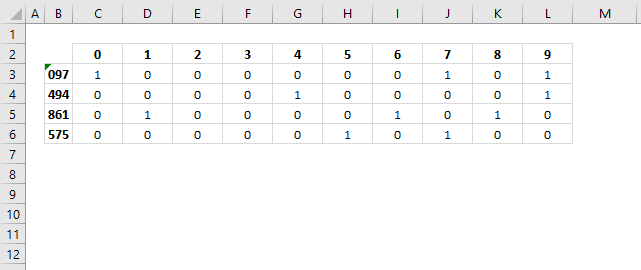#### Step 4 - Merge values column-wise using OR logic

The MMULT function is a capable of aplying OR logic for each column, the result is an array shown in row 7 in the image below.

MMULT(TRANSPOSE(ROW(B3:B6)^0), ISNUMBER(SEARCH({0, 1, 2, 3, 4, 5, 6, 7, 8, 9}, B3:B6))*1)

becomes

MMULT({1, 0, 0, 0, 0, 0, 0, 1, 0, 1;0, 0, 0, 0, 1, 0, 0, 0, 0, 1;0, 1, 0, 0, 0, 0, 1, 0, 1, 0;0, 0, 0, 0, 0, 1, 0, 1, 0, 0})

and returns

{1, 1, 0, 0, 1, 1, 1, 2, 1, 2}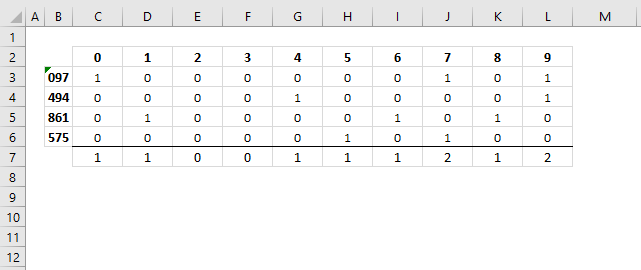#### Step 5 - Replace array values

The IF function returns one value if the logical expression evaluates to True and another value if False, this applies to an array of values as well.

IF(MMULT(TRANSPOSE(ROW(B3:B6)^0), ISNUMBER(SEARCH({0, 1, 2, 3, 4, 5, 6, 7, 8, 9}, B3:B6))*1), {0, 1, 2, 3, 4, 5, 6, 7, 8, 9}, "")

becomes

IF({1, 1, 0, 0, 1, 1, 1, 2, 1, 2}, {0, 1, 2, 3, 4, 5, 6, 7, 8, 9}, "")

0 (zero) equals False and any other value equals True.

IF({1, 1, 0, 0, 1, 1, 1, 2, 1, 2}, {0, 1, 2, 3, 4, 5, 6, 7, 8, 9}, "")

returns

{0,1,"","",4,5,6,7,8,9}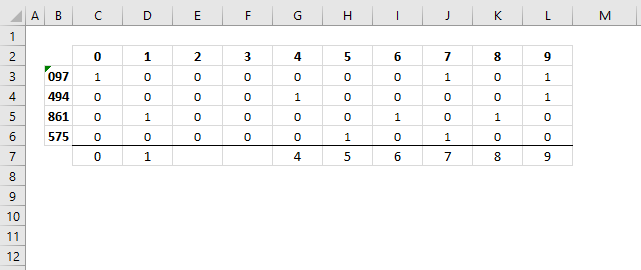The image above shows the following array {0,1,"","",4,5,6,7,8,9} in row 7. It tells you that number two and three are not in cell range C3:C6.

#### Step 6 - Concatenate array values

The TEXTJOIN function is self-explanatory it joins values and is capable of ignoring blank values with any given delimiting character or string.

TEXTJOIN(delimiterignore_emptytext1[text2], ...)

TEXTJOIN("", TRUE, IF(MMULT(TRANSPOSE(ROW(B3:B6)^0), ISNUMBER(SEARCH({0, 1, 2, 3, 4, 5, 6, 7, 8, 9}, B3:B6))*1), {0, 1, 2, 3, 4, 5, 6, 7, 8, 9}, ""))

becomes

TEXTJOIN("", TRUE, {0,1,"","",4,5,6,7,8,9})

and returns "01456789".

#### Step 7 - Shorter formula

The LET function allows you to write shorter formulas by referring to names that contain a repeated expression. Our formula uses this array {0, 1, 2, 3, 4, 5, 6, 7, 8, 9} twice, we can use the LET function and name the array in order to simplify the formula.

LET(name1, name_value1, calculation_or_name2, [name_value2, calculation_or_name3...])

I named the array x and used x in two locations in the formula. This shortens the formula by 17 characters.

LET(x, {0, 1, 2, 3, 4, 5, 6, 7, 8, 9}, TEXTJOIN("", TRUE, IF(MMULT(TRANSPOSE(ROW(B3:B6)^0), ISNUMBER(SEARCH(x, B3:B6))*1), x, "")))

returns "01456789" in cell E2.

#### Will this formula work with blanks?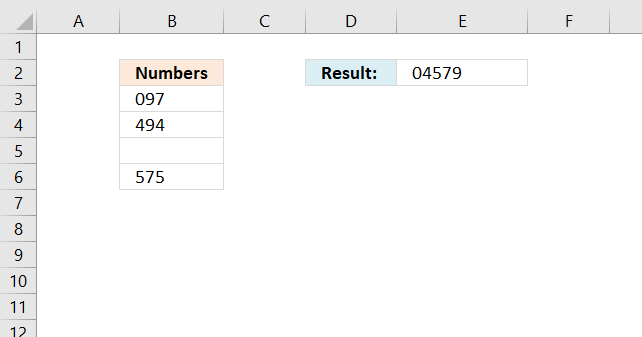Yes, it works fine with blank cells in column B.

#### Can I mix text and numbers in column B?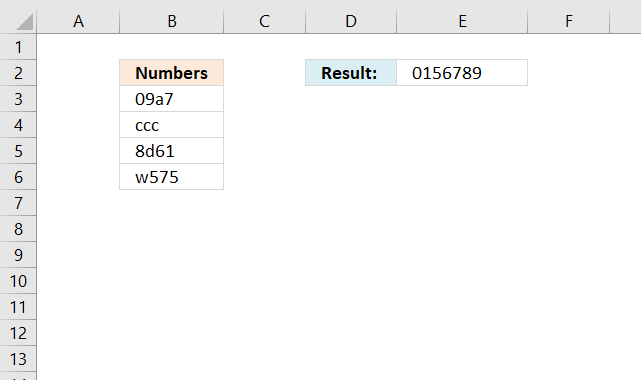Yes, the formula ignores letters and other characters.

#### Can I use values across columns?

No, the formula breaks if you try a multicolumn cell range like B3:C6.

### Get the Excel fileFind-and-sort-numbersv3.xlsx